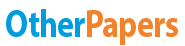# Solubility Product of Lead Chloride

Essay by   •  July 31, 2011  •  Essay  •  1,051 Words (5 Pages)  •  1,700 Views

## Essay Preview: Solubility Product of Lead Chloride

Report this essay
Page 1 of 5

Title : Solubility product of Lead Chloride

Aim : to determine the solubility and the solubility product of lead chloride in pure water

Method/ material : refer to the handout

Result

1. Copy the table below and supply the necessary experimental data with proper calculations

A B

Mass of weighing tray + PbCl2 4.47g 4.66g

Mass of weighing tray after transfer of PbCl2 1.472g 1.66g

Mass of PbCl2 3g 3g

Mass of sintered crucible 30.45g 31.11g

Mass of sintered crucible +residue 31.07g 32g

Mass of residue 0.62g 0.89g

Mass of PbCl2 dissolved 2.38g 2.11g

Amount of PbCl2 dissolved 8.56 x (10^-3) mol 7.59 x (10^-3) mol

Concentration of PbCl2 dissolved 0.0428M 0.038M

 Mass of PbCl2 : (Mass of weighing tray+PbCl2) - (Mass of weighing tray after transfer of PbCl2)

A : 4.47g - 1.472g = 3 g

B : 4.66g - 1.66g = 3 g

 Mass of residue : (Mass of sintered crucible +residue) - (Mass of sintered crucible)

A : 31.07g - 30.45g = 0.62g

B : 32g - 31.11g = 0.89g

 Mass of PbCl2 dissolved : (Mass of PbCl2) - (Mass of residue)

A : 3g - 0.62g = 2.38 g

B : 3g - 0.89g = 2.11g

 Molar weight of PbCl2

Pb : 207.2 g/mol

Cl : 35.45 g/mol

207.2 g/mol + (2 x 35.45 g/mol) = 278.1 g/mol

 Amount of PbCl2 dissolved : Mass of PbCl2 dissolved / Molar weight of PbCl2

A : 2.38g / (278.1g/mol) = 8.56 x (10^-3) mol

B : 2.11g / (278.1g/mol) = 7.59 x (10^-3) mol

 Concentration of PbCl2 dissolved ( in 200ml of distilled water)

A : 8.56 x (10^-3) mol / 200ml x 1L/1000ml = 0.0428 mol/L = 0.0428 M

B : 7.59 x (10^-3) mol / 200ml x 1L/1000ml = 0.03795 mol/L = 0.038M

2. Calculate the Ksp for PbCl2

PbCl2 <-> Pb(2+) + 2 Cl(-)

1 : 1 : 2

Ksp = [Pb(2+)] x [Cl(-)]^2

Ksp of A : [Pb(2+)] = 0.0428

[Cl(-)] = 0.0428 x 2 = 0.0856 M

= [0.0428] x [0.0856]^2

Ksp of A = 3.14 x (10^-4) mol(3) /dm(9)

Ksp of B : [Pb(2+)] = 0.038M

[Cl(-)] = 0.038M x 2 = 0.076 M

= [0.038] x [0.076]^2

Ksp of B = 2.2 x (10^-4) mol(3) / dm(9)

3. Any change (procedure 6)

When we allow the lead chloride to cool to room temperature,

...

...

Download as:   txt (5.7 Kb)   pdf (93.8 Kb)   docx (10.9 Kb)
Continue for 4 more pages »
Only available on OtherPapers.com
Citation Generator

(2011, 07). Solubility Product of Lead Chloride. OtherPapers.com. Retrieved 07, 2011, from https://www.otherpapers.com/essay/Solubility-Product-of-Lead-Chloride/8037.html

"Solubility Product of Lead Chloride" OtherPapers.com. 07 2011. 2011. 07 2011 <https://www.otherpapers.com/essay/Solubility-Product-of-Lead-Chloride/8037.html>.

"Solubility Product of Lead Chloride." OtherPapers.com. OtherPapers.com, 07 2011. Web. 07 2011. <https://www.otherpapers.com/essay/Solubility-Product-of-Lead-Chloride/8037.html>.

"Solubility Product of Lead Chloride." OtherPapers.com. 07, 2011. Accessed 07, 2011. https://www.otherpapers.com/essay/Solubility-Product-of-Lead-Chloride/8037.html.